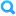## RubricFind Rubric
Keep in mind that 59 students have already been assessed using this rubric. Changing it will affect their evaluations.
Problem Set 5 Rubric
Problem Set 5 Rubric
Criteria Ratings Pts
Least Squares Error deriving set of linear equations in the coefficients
threshold: pts
 4.0 to >3.0 pts Derived set of equations is linear in A,B,C; Derivation is clear and does not skip steps; Derivation starts from correct definition of error. blank 3.0 to >2.0 pts Small logical error in derivation or a few skipped steps; Derived set of equations is linear in A,B,C; _1530 2.0 to >1.0 pts Multiple or large logical errors, incorrect set of equations (nonlinear), incorrect definition of the error; but significant work shown _2430 1.0 to >0.0 pts Answer with no work shown _3246 0.0 to >0 pts No answer blank_2
pts
4.0 pts
--
Plot (b) iv 2nd order polynomial fit
threshold: pts
 3.0 to >2.0 pts Correct graph with both data and polynomial fit plotted _4977 2.0 to >1.0 pts Missing part of graph _557 1.0 to >0.0 pts Graphed by hand without precision _9725 0.0 to >0 pts No graph _4267
pts
3.0 pts
--
Plot (c) iv 3rd order polynomial fit
threshold: pts
 3.0 to >2.0 pts Correct graph with both data and polynomial fit plotted _9454 2.0 to >1.0 pts Missing part of graph _5877 1.0 to >0.0 pts Graphed by hand without precision _6393 0.0 to >0 pts No graph _793
pts
3.0 pts
--
Total Points: 10.0 out of 10.0
 I'll write free-form comments when assessing students Remove points from rubric Don't post Outcomes results to Learning Mastery Gradebook Use this rubric for assignment grading Hide score total for assessment results
 I'll write free-form comments when assessing students Remove points from rubric Don't post Outcomes results to Learning Mastery Gradebook Use this rubric for assignment grading Hide score total for assessment results